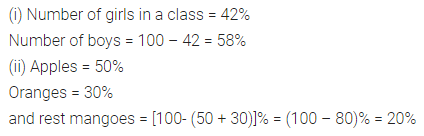ML Aggarwal Class 7 Solutions Chapter 7 Percentage and Its Applications Ex 7.1 for ICSE Understanding Mathematics acts as the best resource during your learning and helps you score well in your exams.

## ML Aggarwal Class 7 Solutions for ICSE Maths Chapter 7 Percentage and Its Applications Ex 7.1

Question 1.
Convert the following percents into fractions in simplest form:
(i) 25%
(ii) 150%
(iii) 7$$\frac { 1 }{ 2 }$$ %
(iv) 33$$\frac { 1 }{ 3 }$$ %
Solution:Question 2.
Convert the following fractions into percents:
(i) $$\frac { 1 }{ 8 }$$
(ii) $$\frac { 5 }{ 4 }$$
(iii) $$\frac { 9 }{ 16 }$$
(iv) $$\frac { 3 }{ 7 }$$
(v) $$\frac { 11 }{ 15 }$$
(vi) 1$$\frac { 3 }{ 8 }$$
Solution: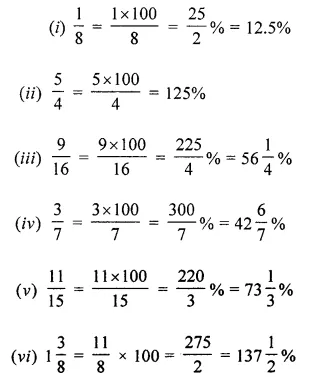Question 3.
(i) 6 students out of 40 students in a class are absent. What percentage of students are absent ?
(ii) Antony secured 384 marks out of 500 marks. Find the percentage of marks secured by Antony.
(iii) A shop has 500 shirts, out of which 15 are defective. What percentage of shirts are defective?
(iv) Vani has a collection of bangles. She has 20 gold bangles and 10 silver bangles. What is the percentage of each type of bangles?
(v) There are 120 voters and 90 of them voted. What percent did not vote?
Solution: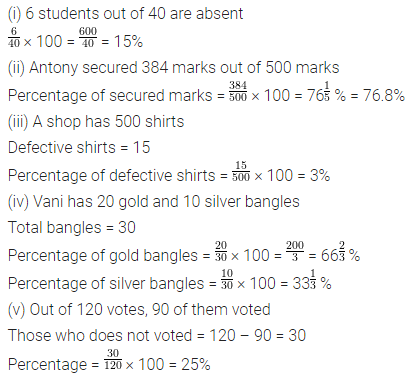Question 4.
Estimate the part of the figure which is shaded and hence find the percentage of the part which is shaded.Solution: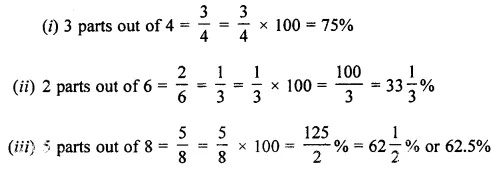Question 5.
Convert the following percentage into ratios in sirup rest form:
(i) 14 %
(ii) 1$$\frac { 3 }{ 4 }$$ %
(iii) 33$$\frac { 1 }{ 3 }$$ %
(iv) 37.5 %
Solution: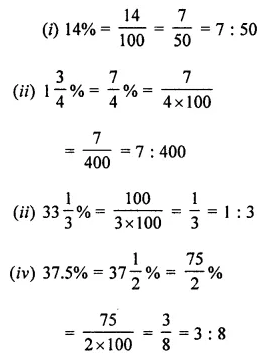Question 6.
Express the following ratios as percentages:
(i) 5 : 4
(ii) 1 : 1
(iii) 2 : 3
(iv) 9 : 16
Solution: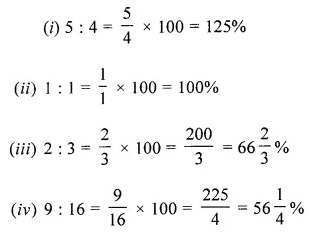Question 7.
An alloy consists of 7 parts of zinc and 33 parts of copper. Find the percentage of copper in the alloy ?
Solution: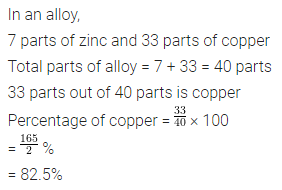Question 8.
Chalk contains calcium, carbon and sand in the ratio 12 : 3 : 10. Find the percentage of carbon in the chalk 12%.
Solution: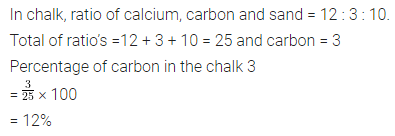Question 9.
If ₹ 2500 is to be divided among Ravi, Raju and Roy, so that Ravi gets two parts, Raju three parts and Roy five parts. How much money will each get? What will it be in percentages?
Solution: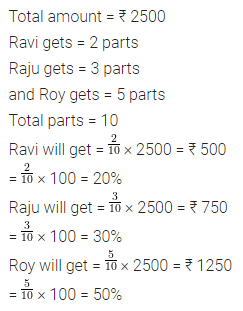Question 10.
Convert the following percentages to decimals:
(i) 28%
(ii) 3%
(iii) 0. 44%
(iv) 37$$\frac { 1 }{ 2 }$$ %
Solution: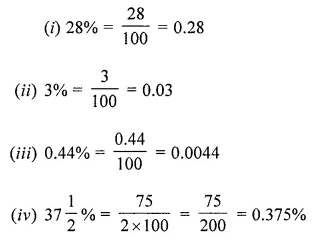Question 11.
Convert the following decimals to percents:
(i) 0.65
(ii) 0.9
(iii) 2.1
(iv) 0.02
Solution: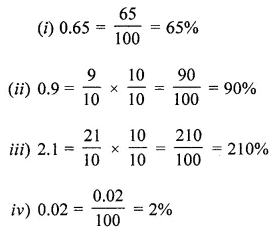Question 12.
(i) If 42% of the students in a class are girls, then what percent of the students in the class are boys?
(ii) We have a basket full of apples, oranges, and mangoes if 50% are apples, 30% are oranges, then what percent are mangoes?
Solution: